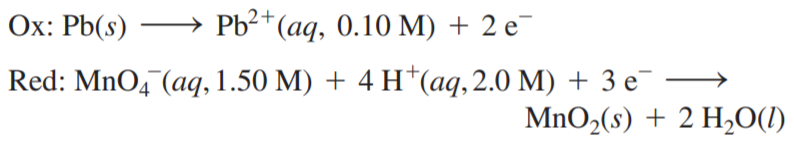×
Get Full Access to Chemistry: A Molecular Approach - 3 Edition - Chapter 18 - Problem 75e
Get Full Access to Chemistry: A Molecular Approach - 3 Edition - Chapter 18 - Problem 75e

×

# An electrochemical cell is based on these twoISBN: 9780321809247 1

## Solution for problem 75E Chapter 18

Chemistry: A Molecular Approach | 3rd Edition

• Textbook Solutions
• 2901 Step-by-step solutions solved by professors and subject experts
• Get 24/7 help from StudySoup virtual teaching assistantsChemistry: A Molecular Approach | 3rd Edition

4 5 1 251 Reviews
15
5
Problem 75E

An electrochemical cell is based on these two half-reactions:Calculate the cell potential at 25 oC.

Step-by-Step Solution:
Step 1 of 3

Step 2 of 3

Step 3 of 3

##### ISBN: 9780321809247

Since the solution to 75E from 18 chapter was answered, more than 245 students have viewed the full step-by-step answer. This textbook survival guide was created for the textbook: Chemistry: A Molecular Approach, edition: 3. The full step-by-step solution to problem: 75E from chapter: 18 was answered by , our top Chemistry solution expert on 02/22/17, 04:35PM. Chemistry: A Molecular Approach was written by and is associated to the ISBN: 9780321809247. This full solution covers the following key subjects: Cell, electrochemical, half, calculate, based. This expansive textbook survival guide covers 82 chapters, and 9454 solutions. The answer to “?An electrochemical cell is based on these two half-reactions: Calculate the cell potential at 25 oC.” is broken down into a number of easy to follow steps, and 16 words.

Unlock Textbook Solution